# Introduction to Pandas for Data Science

The Pandas library is core to any Data Science work in Python. This introduction will walk you through the basics of data manipulating, and features many of Pandas important features.What is Pandas actually, and why is it so famous? Think of Pandas as an Excel sheet, but a next level Excel sheet with more features and flexibility than Excel.

### Why Pandas

There are a lot of reasons to choose pandas, some of them are

• Open Source
• Easy to Learn
• Great Community
• Built on Top of Numpy
• Easy to Analyze and pre-process data in it
• Built-in Data Visualization
• A lot of Built-in functions to help in Exploratory Data Analysis
• Built-in support for CSV, SQL, HTML, JSON, pickle, excel, clipboard and a lot more
• and a lot more

### Installing Pandas

If you are using Anaconda, you will automatically have pandas in it, but for some reason, if you do not have it, just run this command

```conda install pandas

```

If you are not using Anaconda, install via pip by

```pip install pandas

```

Importing

To import pandas, use

```import pandas as pd
import numpy as np

```

It is better to import numpy with pandas to have access to more numpy features, which helps us in Exploratory Data Analysis (EDA).

### Pandas Data Structures

Pandas has two main data structures.

• Series
• Data Frames

Series

Think of Series as a single column in an Excel sheet. You can also think of it as a 1d Numpy array. The only thing that differentiates it from 1d Numpy array is that we can have Index Names.

The basic syntax to create a pandas Series is as follows:

```newSeries = pd.Series(data , index)
```

Data can be of Any type from Python’s dictionary to list or tuple. It can also be a numpy array.

Let’s build a series from Python List:

```mylist = ['Ahmad','Machine Learning', 20, 'Pakistan']
labels = ['Name', 'Career', 'Age', 'Country']
newSeries = pd.Series(mylist,labels)
print(newSeries)

```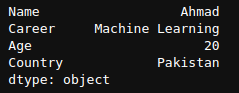Output of newSeries.

It is not necessary to add an index in a pandas Series. In that case, it will automatically start index from 0.

```mylist = ['Ahmad','Machine Learning', 20, 'Pakistan']
newSeries = pd.Series(mylist)
print(newSeries)

```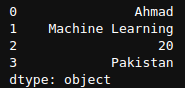Here we can see that the index starts from 0 and goes on till the Series end. Now let’s see how we can create a Series using a Python Dictionary,

```myDict = {'Name': 'Ahmad',
'Career': 'Machine Learning',
'Age': 20,
'Country': 'Pakistan'}
mySeries = pd.Series(myDict)
print(mySeries)

```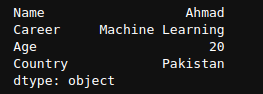Here we can see that we do not have to explicitly pass the index values as they are automatically assigned from the keys in the dictionary.

Accessing data from Series

The normal pattern to access the data from Pandas Series is

```seriesName['IndexName']

```

Let’s take the example of mySeries we created earlier. To get the value of Name, Age, and Career, all we have to do is

```print(mySeries['Name'])
print(mySeries['Age'])
print(mySeries['Career'])

```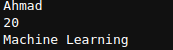Basic Operations on Pandas Series

Let’s create two new series to perform operations on them

```newSeries = pd.Series([10,20,30,40],index=['LONDON','NEWYORK','Washington','Manchester'])
newSeries1 = pd.Series([10,20,35,46],index=['LONDON','NEWYORK','Istanbul','Karachi'])
print(newSeries,newSeries1,sep='\n\n')

```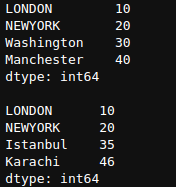Basic Arithmetic operations include +-*/ operations. These are done over-index, so let’s perform them.

```newSeries + newSeries1

```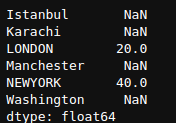Here we can see that since London and NEWYORK index are present in both Series, so it has added the value of both and output of rest is NaN (Not a number).

```newSeries * newSeries1

```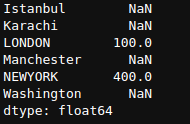```newSeries / newSeries1

```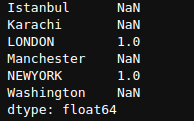If you are familiar with Numpy, you must be aware of the Broadcasting concept. Refer to this link if you are not familiar with the concept of broadcasting.

Now using our newSeries Series, we will see operations performed using the broadcasting concept.

```newSeries + 5

```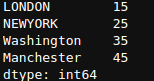Here, it added 5 to every single element in Series newSeries. This is also known as element-wise operations. Similarly, for other operations such as *, /, -, **, and other operators as well. We will see only ** operator, and you should try it for other operators too.

```newSeries ** 1/2

```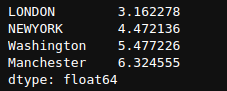Here, we take the element-wise square root of each number. Remember, the square root is any number raised to power 1/2.

Pandas DataFrame

Dataframe is indeed the most commonly used and important data structure of Pandas. Think of a data frame as an excel sheet.

Main ways to create Data Frame are

• Python Dictionary
• ndarray

Let’s take an example of how to create a data frame using a dictionary.

We can create a data frame by passing in a dictionary where each value of the dictionary is a list.

```df1 = {"Name":["Ahmad","Ali",'Ismail',"John"],"Age":  [20,21,19,17],"Height":[5.1,5.6,6.1,5.7]}

```

To convert this dictionary into a data frame, we simply have to call the dataframe function on this dictionary.

```df1 = pd.DataFrame(df1)
df1

```df1

Getting Values from a Column

To get values from a column, we can use this syntax

```#df1['columnname']
#df1.columnname

```

Both of these syntaxes are correct, but we have to be careful about choosing one. If our column name has space in it, then definitely we can not use the 2nd method. We have to use the first method. We can only use the 2nd method when there is no space in the column name.

```df1.Name

```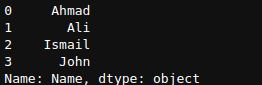df1.Name

Here we can see the values of the column, their index number, name of the column, and datatype of the column.

```df1['Age']

```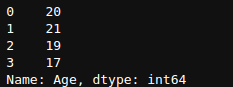df1[‘Age’]

We can see that using both syntaxes returns the column of the data frame where we can analyze it.

Values of Multiple Columns

To get values of Multiple columns in a data frame, pass the name of columns as a list.

```df1[["Name","Age"]]

```df1[[“Name”,”Age”]]

We can see that it returned the dataframe with two columns Name and Age.

### Important functions of Dataframe in Pandas

Let’s explore important functions of DataFrame by using a dataset known as ‘Titanic’. This data set is commonly Available online, or you can get it at Kaggle.

Pandas has good built-in support to read data of various types, including CSV, fether, excel, HTML, JSON, pickle, SAS, SQL, and many more.

The common syntax to read data is

```# pd.read_fileExtensionName("File Path")

```

CSV

To read data from a CSV file, all you have to do is to use pandas read_csv function.

```df = pd.read_csv('Path_To_CSVFile')

```

Since titanic is also available in CSV format, so we will read it using read_csv function. When you download the dataset, you will have two files named train.csv and test.csv, which will help in testing machine learning models, so we will only focus on the train_csv file.

```df = pd.read_csv('train.csv')

```

Now df is automatically a data frame. Let’s explore some of its functions.

If you print your dataset normally, it will show a complete dataset, which may have a million rows or columns, which is hard to see and analyze. df.head() function allows us to see the first ’n’ rows of the data set (by default 5) so that we can make a rough estimate of our dataset, and what important functions to apply on it next.

```df.head()

```df.head()

Now we can see our columns in the data set, & their values for the first 5 rows. Since we haven’t passed any value, so it is showing the first 5 rows.

tail()

Similar to the head function, we have a tail function that shows last n values.

```df.tail(3)

```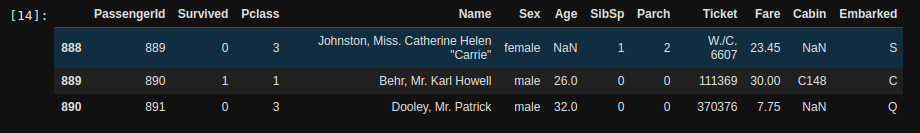df.tail(3)

We can see the last 3 rows from our data set, as we passed df.tail(3).

shape()

The shape() is one another important function to analyze the shape of the dataset, which is pretty helpful when we are making our machine learning models, and we want our dimensions to be exact.

```df.shape()

```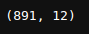df.shape()

Here we can see that our output is (891,12), which is equal to 891 rows and 12 columns, which means that in total, we have 12 features or 12 columns and 891 rows or 891 examples.

Previously when we used the df.tail() function, the index number of our last column was 890 because our index started from 0, not from 1. If the index number started from 1, then we would have an index number of the last column as 891.

isnull()

This is another important function that is used to find the null values in a dataset. We can see in previous outputs that some values are NaN, which means “Not a Number”, and we have to deal with these missing values to get good results. isnull() is an important function to deal with these null values.

```df.isnull().head()

```

I am using the head function so that we can see the first 5 examples, not the whole data set.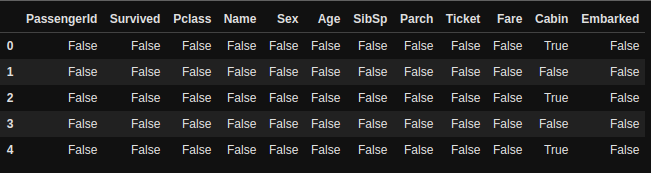Here, we can see that some values in “Cabin” columns are True. True means that the value is NaN or missing. We can see that this is unclear to see and understand, so we can use the sum() function to get more detailed info.

sum()

The sum function is used to sum all the values in a data frame. Remember that True meaning 1 and False meaning 0, so to get all the True values returned by isnull() function, we can use sum() function. Let’s check it out.

```df.isnull().sum()

```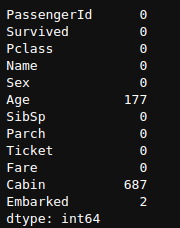df.isnull().sum()

Here, we can see that only missing values are in columns of “Age”, “Cabin”, & “Embarked”.

info()

The info function is also a commonly used pandas function, which “Prints a concise summary of a DataFrame.”

```df.info()

```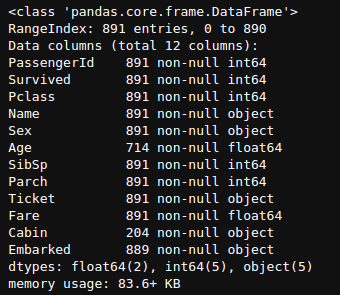df.info()

Here, we can see that it tells us how many non-null entities we have, such as in-case of Age, we have 714 Non-Null float64 type entities. It also tells us about memory usage, which is 83.6 KB in this case.

describe()

Describe is also a super useful function to analyze the data. It tells us about the descriptive statistics of a data frame, including those that summarize the central tendency, dispersion, and shape of a dataset’s distribution, excluding values.

```<df.describe()

```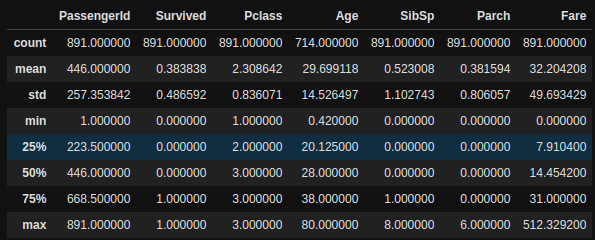df.describe()

Here we can see some important statistical analysis of each column, including the mean, standard deviation, minimum value, and much more. Read more about it in its documentation.

### Boolean Indexing

This is also one of the important and widely used concepts both in Numpy and Pandas.

As the name suggests, we index using boolean variables, i.e., True and False. If the index is True, show that row, and if the Index is False, do not show that row.

It helps us a lot when we are trying to extract important features from our dataset. Let’s take an example where we only want to see the entries where “Sex” is “male”. Let’s see how we are going to approach this.

```df["Sex"]=="male"

```

This is going to return a Series of Boolean values True and False, where True is the row where “Sex” is “male” else False.

In order to see the first 10 results only, I can use the head function as

```(df["Sex"]=="male").head(10)

```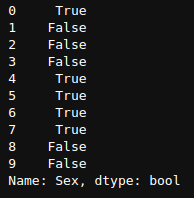(df[“sex”]==”male”).head(10)

Now, in order to see the complete dataframe with only those rows where “Sex” is “male”, we should pass df[“Sex”]==”male” in dataframe to get all the results where “Sex” is “male”.

```df[df["Sex"]=="male"].head(5)

```Here, we can see that all the results we have are those where Sex is Male.

Now let’s derive some useful information using Boolean indexing.

In order to get rows based on multiple conditions, we use parenthesis “()” and “&,|,!=” signs between multiple conditions.

Let’s find out what is the percentage of all the male passengers who have survived.

```df[(df["Sex"]=="male") & (df["Survived"]==1)]

```Give this code a second to read and understand what is happening. We are collecting all the rows from the dataframe where df[“Sex”] == “male” and df[“Survived”]==1. The returned value is a dataframe with all the rows of male passengers who survived.

Let’s find out the percentage of male passengers survived.

Now, the formula for the percentage of male passengers who survived is Total Number of Male Survived / Total Number of Male Passengers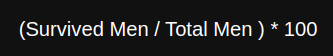In pandas, we can write this as

```men = df[df['Sex']=='male']['Survived']
perc = sum(men) / len(men) * 100

```

Let’s break this code step by step.

df[‘Sex’]==’male’ will return a boolean Series of examples where sex is male.

df[df[“Sex”]==”male”] will return the complete data frame with all examples where “Sex” is “male”.

men = df[df[‘Sex’]==’male’][‘Survived’] will return the “Survived” column of data frame of all the passengers who are male.

sum(men) will sum all the men who survived. As it is a Series of 0 and 1. len(men) will return the Number of Total men.

Now put these in the formula given above and we will find the percentage of all the men survived in Titanic which is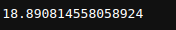18%!!!!!. Yes, only 18% of men in our data set have survived the Titanic disaster.

Similarly, we can code it for females, which I won’t, but it is your task, we find out that 74 % of female passengers have survived this disaster.

This brings us to the end of this article. Now obviously there are tons of other important functions in Pandas which are very important such as groupby, apply, iloc, rename, replace etc. I recommend you to check the “Python for Data Analysis” book by Wes, who is the creator of this Pandas library.

Also, check out this cheat sheet by Dataquest for quick reference.

Related: